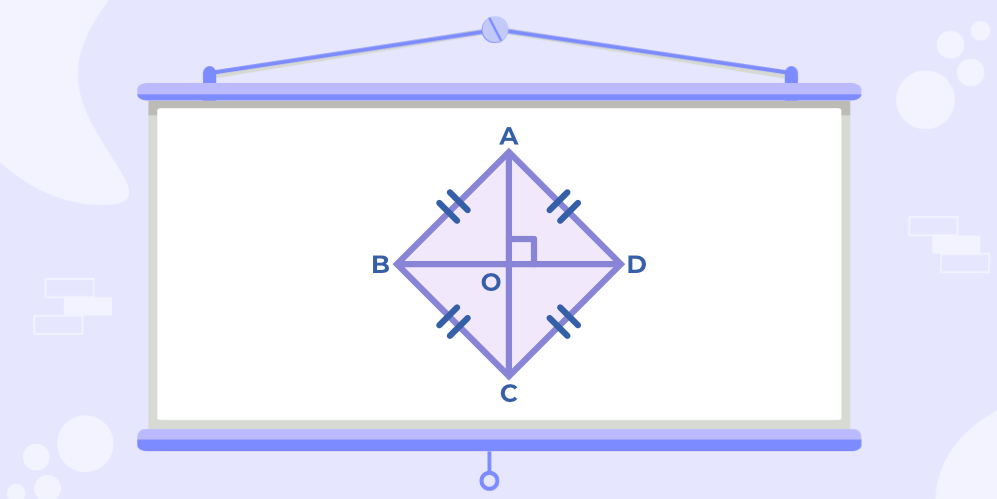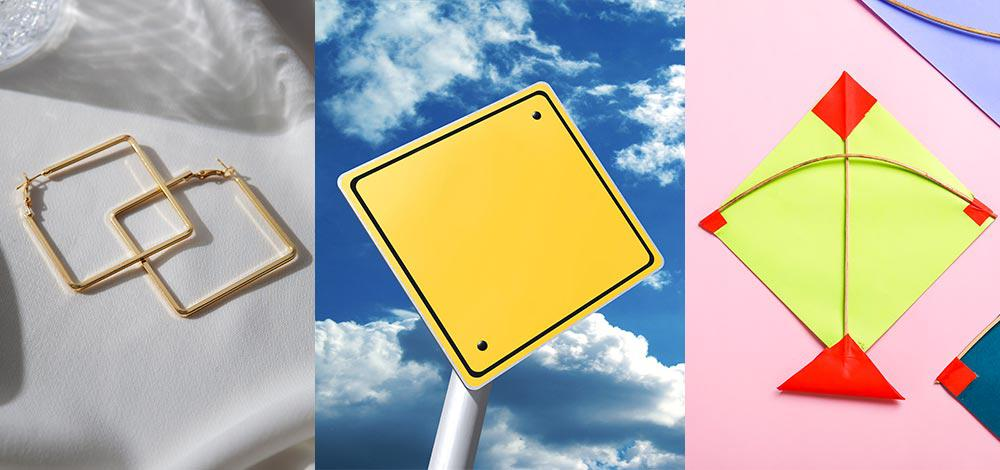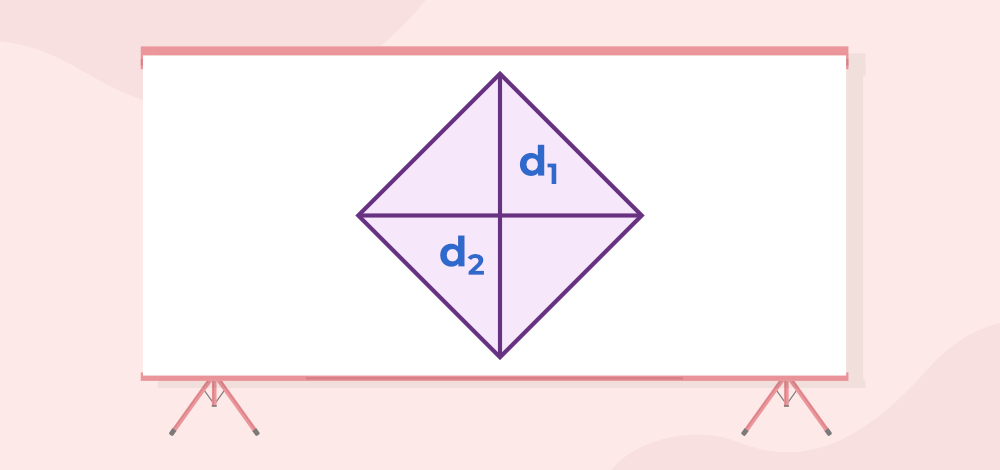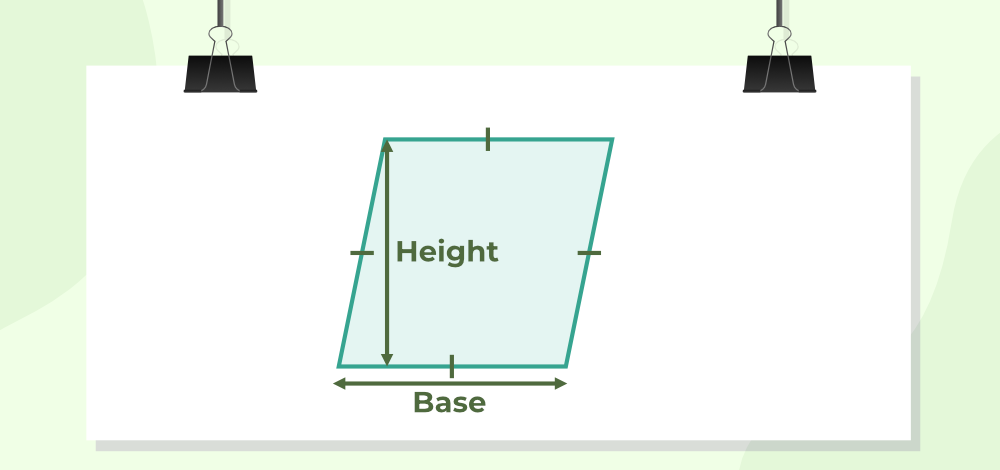Open In App

# Rhombus

A rhombus is a quadrilateral with all four sides equal and opposite sides parallel to each other, also its opposite angles are equal. Any rhombus can be considered a parallelogram, but not all parallelograms are considered rhombus. Similarly, all squares can be considered rhombuses, but not all rhombuses are considered squares. Diagonals of a rhombus are orthogonal bisectors of each other.

It is important to note that all squares are rhombuses, but not all rhombuses are squares. This is because a square is a special type of rhombus that has all four sides equal in length and all four angles equal to 90 degrees. However, a rhombus can have angles that are not equal to 90 degrees.

In this article, we will discuss what is a rhombus, rhombus formula, area of the rhombus, rhombus properties, examples, etc.

Table of Content

## What is a Rhombus?

A rhombus is a special case of a quadrilateral known as a parallelogram. where the adjacent sides are equal in length and also the diagonals bisect each other at right angles. We can also state that a rhombus is actually a square only when all its angles are equal to 90 degrees. The figure below shows a rhombus shape where AB = BC = CD = DA and the diagonals AC and BD bisect each other at a right angle, confirming its classification as a quadrilateral.

### Rhombus Definition:Rhombus

## Properties of a Rhombus

The rhombus, classified as a special type of parallelogram, exhibits all the defining properties of parallelograms. Here are a few notable properties of the rhombus:

• All the sides of a rhombus are equal. In fact, it is just a parallelogram with equal adjacent sides.
• All Rhombus has two diagonals, which connect the pairs of opposite vertices. A rhombus is symmetrical along both its diagonals. The diagonals of a rhombus are perpendicular bisectors to each other.
• In the event of all the angles of a rhombus are equal, it is called a square.
• The diagonals of a rhombus would always bisect each other at a 90 degrees angle.
• Not only do the diagonals bisect each other, but they also bisect the angles of a rhombus.
• The two diagonals of a rhombus divide it into four right-angled congruent triangles.
• There cannot be a circumscribing circle around a Rhombus.
• It is impossible to have an inscribing circle inside a rhombus.

## Rhombus Examples

Rhombus is a very common shape and can be seen in a variety of objects which we use in our daily lives. Various Rhombus-shaped objects are Jewelry, Kites, Sweets, Furniture, etc.Rhombus Examples

## Rhombus Formula

There are some important formulas related to Rhombus, which are as follows:

• Area
• perimeter

### Area of Rhombus

The area of the Rhombus is the space enclosed by all four boundaries of the Rhombus it is measured in unit squares. There are two ways of finding Areas of a Rhombus which are discussed below

#### 1.) Area of Rhombus when both Diagonals are given

The area of the rhombus is the region covered by it in a two-dimensional plane. The formula for the area is equal to the product of the diagonals of the rhombus divided by 2. It can be represented as:

Area of Rhombus = 1/2(d1 × d2)  Sq. unit

where d1 and d2 are diagonals of a rhombus.Area of Rhombus

#### 2.) Area of Rhombus when Base and Altitude are given

When the Base and Altitude of a Rhombus are given then the formula calculates its area:

Area of Rhombus = Base × HeightArea of Rhombus

### Perimeter of a Rhombus

Perimeter of a rhombus is defined as the sum of all its sides. Since all the sides of a rhombus are equal in length, it can be said that the Perimeter of a Rhombus is four times the length of one side. Thus, if s denotes the length of a side of a rhombus, its perimeter = 4s.

Perimeter of Rhombus = 4×s

where s is the side of Rhombus

## Is a Rhombus a Square?

Rhombus and Square both are Equilateral Quadrilaterals, i.e. both have equal all four sides. The difference between them is that all angles in a square are equal and right angles, but on the other hand, all the angles of a rhombus need not be equal.

Hence, Rhombus with right angles is called a Square.

Thus, “Every Square is a Rhombus but all Rhombus are not Squares.”

For more, check out :

## Solved Examples on Rhombus

### Example 1: MNOP is a rhombus. If diagonal MO = 29 cm and diagonal NP = 14 cm, What is the area of rhombus MNOP?

Solution:

Area of a rhombus = (d1)(d2)/2

Substituting the lengths of diagonals in the above formula, we have:

A = (29)(14)/2 = 406/2 = 203 cm2

Area of rhombus MNOP = 203 cm2

### Example 2: ABCD is a rhombus. The perimeter of ABCD is 40, and the height of the rhombus is 12. What is the area of ABCD?

Solution:

Perimeter = 40 cm

Perimeter = 4 × side

40 = 4×side

⇒ side(base) = 10 cm

and height = 12 cm (given)

Now, Area of Rhombus = base × height

⇒ Area = 10×12 = 120 cm2

Thus, Area of rhombus ABCD is equal to 120 cm2

### Example 3: Find the area of a rhombus with diagonal lengths of (2x+2) and (4x+4) units.

Solution:

We know, Area of a rhombus = (d1)(d2)/2

Substituting the lengths of diagonals in the above formula, we have:

A =⇒ A =⇒ A =⇒ A = (4x2 + 8x + 4) unit2

### Example 4: Find the area of a rhombus if its diagonal lengths arecm andcm.

Solution:

We know, Area of a rhombus = (d1)(d2)/2

Substituting the lengths of diagonals in the above formula, we have:

A =⇒ A =cm2

## FAQs on Rhombus

### 1. What is Rhombus in Geometry?

A rhombus is a 2-D shape with four sides hence termed as a quadrilateral. It has two diagonals that bisect each other at right angles.

### 2. What shape is a rhombus?

A rhombus is a type of quadrilateral shape with four sides of equal length.

### 3. Are all 4 sides of a rhombus equal?

Yes, all four sides of a rhombus are equal in length.

### 4. What are the 4 properties of a rhombus?

The four properties of a rhombus are:

• all four sides are of equal length,
• opposite angles are equal in measure,
• diagonals bisect each other at right angles, and
• consecutive angles are supplementary.

### 5. Is a rhombus a square?

A rhombus becomes a square only when all four angles are equal to 90 degrees.

### 6. What are the 8 properties of a rhombus?

The eight properties of a rhombus are:

• all four sides are of equal length,
• opposite angles are equal in measure,
• diagonals bisect each other at right angles,
• consecutive angles are supplementary,
• diagonals are of equal length,
• the sum of the squares of the four sides is equal to the sum of the squares of the two diagonals,
• the area is equal to half the product of the diagonals, and
• the perimeter is equal to four times the length of one side.

### 7. Are rhombus diagonals equal?

Yes, the diagonals of a rhombus are of equal length.

### 8. What shape has 4 equal sides and equal-length diagonals?

A shape with 4 equal sides and equal length diagonals is a square.